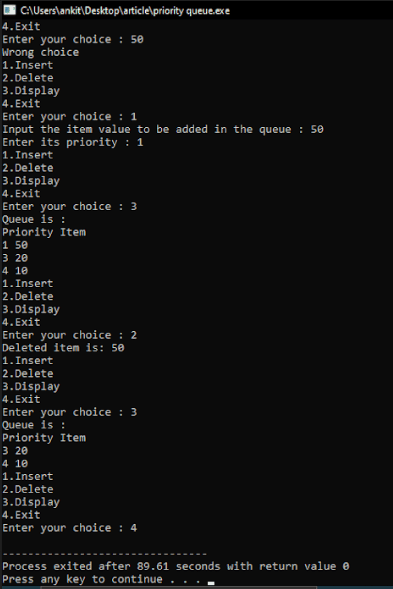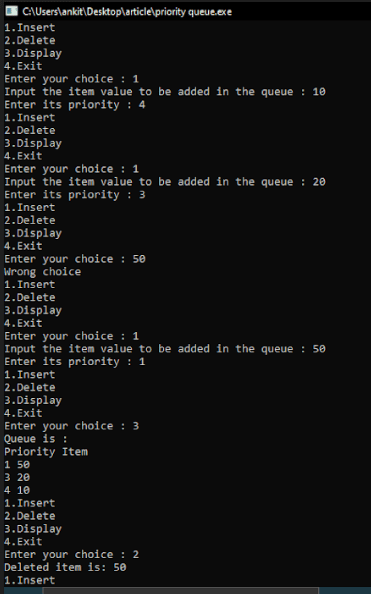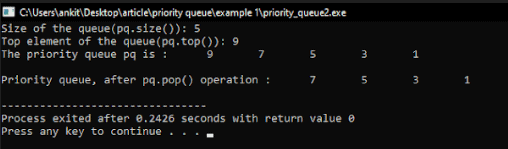# Priority Queue in C++: The Complete Guide

The queue is a data structure based on FIFO (First In First Out), i.e., where insertion is placed at the rear and deletion at the front. A priority Queue is a particular type of queue. Priority Queue doesn’t follow the concept of FIFO like Queue.

## Priority Queue in C++

Priority Queue in C++ is implemented as container adaptors that use the encapsulated object of a specific container class as its underlying container. Each element in the Priority queue is associated with some priority. The deletion of an element takes place based on priority.

The first item to be removed from the queue is an element with the highest priority. Items with the same priority are sorted based on FIFO. These are contained in the STL library of the C++ library. This sort the highest element in the front and others in decreasing order. These have a specific set of member functions to access their elements.

## Basic operations of Priority Queue

### insert (item, priority)

It inserts an item with its priority in the priority queue.

### getHighestPriority()

Returns an item with the highest priority in the queue.

### delete ()

Removes the item with the highest priority and gives the item removed.

### isEmpty()

It checks whether the queue is empty or not.

### isFull()

It checks whether the queue is full or not.

## Advantages of the priority queue

Nodes are given weight, which allows them to move towards the head of the queue rather than being at the tail of the queue as would happen in the regular queue.

## Disadvantages of the priority queue

Insertion takes now doesn’t take a constant time like queue because we also have to apply insertion sort to insert the element based on their priority.

Implementation through the linked list helps to achieve constant time for insertion.

## Algorithm or Pseudocode

```Insert (item, priority)
First element to insert: Insert (10,1)
Second element to insert:Insert (30,3)
Third element to insert: Insert (20,2)
Fourth element to insert:Insert (40,4)
Queue after insertion: 40 30 20 10
Delete ()
First deletion: 40
Queue after deletion: 30 20 10
Insert (50,4)
Insert (60,4)
Queue after insertion :50 60 30 20 10
```

Here we can see that the elements are inserted based on their priority.

The highest priority element is in the front of the queue. Therefore, on deletion, the first queue element is removed from the queue. After that, items with the same priority are sorted based on the FIFO.

## Program of Priority Queue In C++

Example 1: Make a queue using the STL method and perform essential functions.

```#include <iostream>
#include <conio.h>
#include <cstdio>
#include <cstring>
#include <cstdlib>

using namespace std;

struct n // node declaration
{
int priority;
int info;
struct n *next;
};
class Priority_Queue
{
private:
n *f;

public:
Priority_Queue()
{

f = NULL;
}
void insert(int i, int p)
{
n *t, *q;
t = new n;
t->info = i;
t->priority = p;
if (f == NULL || p < f->priority)
{
t->next = f;
f = t;
}
else
{
q = f;
while (q->next != NULL && q->next->priority <= p)
q = q->next;
t->next = q->next;
q->next = t;
}
}
void delet()
{
n *t;
if (f == NULL) //if queue is null
cout << "Queue Underflow\n";
else
{
t = f;
cout << "Deleted item is: " << t->info << endl;
f = f->next;
free(t);
}
}
void show() //print queue {
{
n *ptr;
ptr = f;
if (f == NULL)
cout << "Queue is empty\n";
else
{
cout << "Queue is :\n";
cout << "Priority Item\n";
while (ptr != NULL)
{
cout << ptr->priority << " " << ptr->info << endl;
ptr = ptr->next;
}
}
}
};
int main()
{
int c, i, p;
Priority_Queue pq;
do
{
cout << "1.Insert\n";
cout << "2.Delete\n";
cout << "3.Display\n";
cout << "4.Exit\n";
cout << "Enter your choice : ";
cin >> c;
switch (c)
{
case 1:
cout << "Input the item value to be added in the queue : ";
cin >> i;
cout << "Enter its priority : ";
cin >> p;
pq.insert(i, p);
break;
case 2:
pq.delet();
break;
case 3:
pq.show();
break;
case 4:
break;
default:
cout << "Wrong choice\n";
}
} while (c != 4);

return 0;
}```

See the output.Example 2: Make a queue using a link list and perform essential functions.

```#include <iostream>
#include <queue>

using namespace std;

void displaypq(priority_queue<int> pq)
{
priority_queue<int> pqueue = pq;
while (!pqueue.empty())
{
cout << '\t' << pqueue.top();
pqueue.pop();
}
cout << '\n';
}

int main()
{
priority_queue<int> pq;
pq.push(1);
pq.push(3);
pq.push(5);
pq.push(7);
pq.push(9);

cout << "Size of the queue(pq.size()): " << pq.size();
cout << "\nTop element of the queue(pq.top()): " << pq.top();
cout << "\nThe priority queue pq is : ";
displaypq(pq);

cout << "\nPriority queue, after pq.pop() operation : ";
pq.pop();
displaypq(pq);

return 0;
}```

See the output.## Conclusion

Priority queues are container adapters designed explicitly so that the first element of the queue is the greatest of all items in the queue, and elements are in non-increasing order.

That’s it for this tutorial.

## Recommended Posts

Queues in C++

Deque in C++

C++ Functions

C++ Array

C++ Iterators

Categories C++

This site uses Akismet to reduce spam. Learn how your comment data is processed.